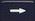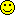# KhiCAS Ableitungen

Ableitung Trinkgeld

7 replies to this topic

### #1naluca

naluca

Newbie

•• Members
•• 4 posts

• Calculators:
Casio fx-CG50

Posted 23 February 2023 - 09:23 PM

Hallo,

ich habe  mir Khicas auf meinen Taschenrechner (fx-CG50) heruntergeladen, um  von einer Funktion die erste Ableitung zu bilden. Ich weiß nicht genau, wie ich jetzt die Funtkion eintippe und  der Taschenrechner mit die Ableitung anzeigt bzw auch am besten direkt zusammenfasst. Falls sich jemand damit auskennt, gerne bitte melden. Als Belohnung gebe ich auch ein kleines Trinkgeld.

Vielen lieben DANK!

### #2HappyCalc

HappyCalc

•• Members
•• 63 posts
• Gender:Male
• Location:Germany
• Interests:Programming, On-Calc Programming(C.Basic), Gaming, Origami, Eating Chocolate

• Calculators:
fx-9860GII-2
fx-CG50

Posted 24 February 2023 - 12:38 PM

In KhiCAS you can either enter expressions in inline notation or mathematical notation.
KhiCAS will normally start in the inline mode. But you can enter the mathematical mode by pressing (view).

As an example, I will use 2x³ + x²

To create a function, you can either enter

```<expression>=><name>
2*x^3+x^2=>f
```

or

```<name>:=<expression>
f:=2*x^3+x^2
```

or

```<name>(<variable>):=<expression>
f(x):=2*x^3+x^2
```

then you can enter

```<expression>'
f'
```

or if you used an other variable than x

```diff(<expression>,<variable>)
diff(f,x)
```

that will result in

```2*3*x^2+2*x
```

to simplify this, you can use

```<expression>=>/
```

or

```simplify(<expression>)
```

then you'll get

```6*x^2+2*x
```

which is the derivative of 3x^3+2x^2. Great!

To sum up:

```f:=3*x^2+x^2
fd:=f'=>/
```

fd will contain the derivative now.

If you used

```f(x):=2*x^3+x^2
```

results will look different:

```(x)->6*x^2+2*x
```

the (x)->... means, that you can use f(x) like a normal function:

You can calculate f(5) by just typing it in.
If you didn't use f(x):=..., you can calculate f(5) by typing

```f(x=5)
```

You can enter the arrow '=>' by pressing thebutton, the apostrophe ' by pressing (calc) . The only way i know to get the colon ':' is by pressing and selecting it by hand. (There was another way in an earlier version, but I guess it was removed).

I hope that helps!(And I don't need a tip, I'm happy if I can help people with calculator stuff)

• naluca and like this

### #3naluca

naluca

Newbie

•• Members
•• 4 posts

• Calculators:
Casio fx-CG50

Posted 24 February 2023 - 10:59 PM

In KhiCAS you can either enter expressions in inline notation or mathematical notation.
KhiCAS will normally start in the inline mode. But you can enter the mathematical mode by pressing (view).

As an example, I will use 2x³ + x²

To create a function, you can either enter

```<expression>=><name>
2*x^3+x^2=>f
```

or

```<name>:=<expression>
f:=2*x^3+x^2
```

or

```<name>(<variable>):=<expression>
f(x):=2*x^3+x^2
```

then you can enter

```<expression>'
f'
```

or if you used an other variable than x

```diff(<expression>,<variable>)
diff(f,x)
```

that will result in

```2*3*x^2+2*x
```

to simplify this, you can use

```<expression>=>/
```

or

```simplify(<expression>)
```

then you'll get

```6*x^2+2*x
```

which is the derivative of 3x^3+2x^2. Great!

To sum up:

```f:=3*x^2+x^2
fd:=f'=>/
```

fd will contain the derivative now.

If you used

```f(x):=2*x^3+x^2
```

results will look different:

```(x)->6*x^2+2*x
```

the (x)->... means, that you can use f(x) like a normal function:

You can calculate f(5) by just typing it in.
If you didn't use f(x):=..., you can calculate f(5) by typing

```f(x=5)
```

You can enter the arrow '=>' by pressing thebutton, the apostrophe ' by pressing (calc) . The only way i know to get the colon ':' is by pressing and selecting it by hand. (There was another way in an earlier version, but I guess it was removed).

I hope that helps!(And I don't need a tip, I'm happy if I can help people with calculator stuff)

Vielen Dank! Ich habe deine Schritte sehr gut verstanden. Weiß du, wie ich das alles also erste Ableitung inklusive zusammenfassen in einen Befehl machen kann den ich direkt auswählen kann (eventuell im Menü)? Falls das nicht geht, wollte ich dich fragen, ob man die Funktionen auch noch besser zusammenfassen/ vereinfach kann mit Klammern. Ein sehr einfaches  Beispiel: f(x)= x^3  --> f(x)= x(x^2)

### #4HappyCalc

HappyCalc

•• Members
•• 63 posts
• Gender:Male
• Location:Germany
• Interests:Programming, On-Calc Programming(C.Basic), Gaming, Origami, Eating Chocolate

• Calculators:
fx-9860GII-2
fx-CG50

Posted 25 February 2023 - 03:54 PM

You can always calculate the derivative of the function directly like that:

```(2*x^3+x^2)'=>/
```

If you instead of simplifying the result want to factorize it, use =>* instead:

```f'=>*
(2*x^3+x^2)'=>*
```

which will result in

```2*x*(x^2+1)
```

### #5pan.gejt

pan.gejt

Casio Freak

•• Members
•• 247 posts
• Gender:Male
• Location:CZ

• Calculators:
.

Posted 25 February 2023 - 09:17 PM

You can enter the assignment operator ":=" by pressing shiftbutton

Edited by pan.gejt, 25 February 2023 - 09:18 PM.

### #6naluca

naluca

Newbie

•• Members
•• 4 posts

• Calculators:
Casio fx-CG50

Posted 28 February 2023 - 08:34 PM

You can always calculate the derivative of the function directly like that:

```(2*x^3+x^2)'=>/
```

If you instead of simplifying the result want to factorize it, use =>* instead:

```f'=>*
(2*x^3+x^2)'=>*
```

which will result in

```2*x*(x^2+1)
```

Hallo, vielen Dank. Jedoch zeigt der mir bei einer E Funktion häufig einen Bruch an, geht das auch ohne Bruch?

### #7HappyCalc

HappyCalc

•• Members
•• 63 posts
• Gender:Male
• Location:Germany
• Interests:Programming, On-Calc Programming(C.Basic), Gaming, Origami, Eating Chocolate

• Calculators:
fx-9860GII-2
fx-CG50

Posted 11 March 2023 - 05:26 PM

Yes, you can use the approx() function to calculate an approximate result:

`approx(e^3)`
`20.0855369`

You can find it in the menu "real"

### #8critor

critor

Casio Fan

•• Members
•• 38 posts

• Calculators:
Graph 25+ (fx-7400G)
Graph 25+Pro (fx-7400GII)
Graph 35+ (fx-9750G)
Graph 35+USB (fx-9750GII)
Graph 65 (?)
Graph 85 (fx-9860G)
Graph 75 (fx-9860GII)
Graph 95 (fx-9860GII SD)

Posted 12 March 2023 - 08:14 PM

Hallo @naluca.

To get a summary of everything, maybe you could try :

```tabvar(2*x^3+x^2)
```But tabvar is only available if you installed the full version of KhiCAS for the fx-CG50 :

https://www-fourier....io/khicasio.zip

#### 0 user(s) are reading this topic

0 members, 0 guests, 0 anonymous users